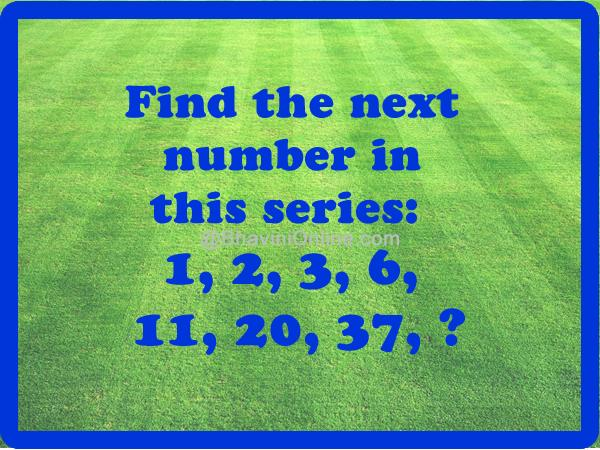# What Comes Next In This Sequence: 1, 2, 3, 6, 11, 20, 37, ?

Check the sequence in this math riddle & answer the question.

#### 1, 2, 3, 6, 11, 20, 37, ?So were you able to solve the riddle? Leave your answers in the comment section below.

## One Reply to “What Comes Next In This Sequence: 1, 2, 3, 6, 11, 20, 37, ?”

1. tanweer ansari says:

not impressed…
it has multiple solutions….

1-2-3-6-11-20-37

now see this…

0+1+2 = 3
1+2+3 = 6
2+3+6 = 11
3+6+11 = 20
6+11+20= 37

so

it can also be 11+20+37 = 68…